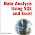## Saturday, May 17, 2008

### The Agent Problem: Sampling From A Finite Population

A drawer is filled with socks and you remove eight of them randomly. Four are black and four are white. How confident are you in estimating the proportion of white and black socks in the drawer?

The standard statistical approach is to assume that the number of socks in the drawer is infinite, and to use the formula for the standard error of a proportion: SQRT([proportion] * [(1 - [proportion])/[number taken out]) or, more simply, SQRT(p*q/n). In this case, the standard error is SQRT(0.5*0.5/8) = 17.7%

However, this approach clearly does not work in all cases. For instance, if there are exactly eight socks in the drawer, then the sample consists of all of them. We are 100% sure that the proportion is exactly 50%.

If there are ten socks in the drawer, then the proportion of black socks ranges from 4/10 to 6/10. These extremes are within one standard error of the observed average. Or to phrase it differently, any reasonable confidence interval (80%, 90%, 95%) contains all possible values. The confidence interval is wider than what is possible.

What does this have to do with business problems? I encountered essentially the same situation when looking at the longitudinal behavior of patients visiting physicians. I had a sample of patients who had visited the physicians and was measuring the use of a particular therapy for a particular diagnosis. Overall, about 20-30% of all patients where in the longitudinal data. And, I had pretty good estimates of the number of diagnoses for each physician.

There are several reasons why this is important. For the company that provides the therapy, knowing which physicians are using it is important. In addition, if the company does any marketing efforts, they would like to see how they perform. So, the critical question is: how well does the observed patient data characterize the physician behavior.

This is very similar to the question posed earlier. If the patient data contains eight new diagnoises and four start on the therapy of interest, how confident am I that the doctor is starting 50% of new patients on the therapy?

If there are eight patients in total, then I am 100% confident, since all of them managed to be in my sample. On the other hand, if the physician has 200 patients, then the statistical measures of standard error are more appropriate.

The situation is exacerbated by another problem. Although the longitudinal data contains 20%-30% of all patients, the distribution over the physicians is much wider. Some physicians have 10% of their patients in the data and some have 50% or more.

The solution is actually quite simple, but not normally taught in early statistics or business statistics courses. There is something called the finite population correction for exactly this situation.
`[stderr-finite] = [stderr-infinite]*fpcfpc = SQRT(([population size]- [sample size])/([population size] - 1))`
So, we simply adjust the standard error and continue with whatever analysis we are using.

There is one caveat to this approach. When observed proportion is 0% or 100%, then the standard error will always be 0, even with the correction. In this case, we need to have a better estimate. In practice, I add or subtract 0.5 from the proportion to calculate the standard error.

This problem is definitely not limited to physicians and medical therapies. I think it becomes an issue in many circumstances where we want to project a global number onto smaller entities.

So, an insurance company may investigate cases for fraud. Overall, they have a large number of cases, but only 5%-10% are in the investigation. If they want to use this information to understand fraud at the agent level, then some agents will have 1% investigated and some 20%. For many of these agents, the correction factor is needed to understand our confidence in their customers' behavior.

The problem occurs because the assumption of an infinite population is reasonable over everyone. However, when we break it into smaller groups (physicians or agents), then the assumption may no longer be valid.

1.Thanks for the clear, understandable explanation of finite populations and the fpc. Do you have suggestions for further reading? I do work in which I need to analyze effects of small populations.

Why do you "add or subtract 0.5 from the proportion to calculate the standard error" hwen the proportion is 0 or 1? Is there a statistical reason or is it an arbitrary correction?

2.I haven't actually found any good sources for this, although you can search "finite population correction factor on the web". It is also mentioned on the wikipedia page for margin of error (http://en.wikipedia.org/wiki/Margin_of_Error) under the heading "Population Adjustment".

When I faced this problem, I asked several statisticians. Surpringly, the one who was able to answer had a masters in statistics and had specialized in surveys. Perhaps this is not so surprising, but several other PhDs did not know the answer.

I haven't yet found a proof of the finite correction factor.

As for your second question, I need the adjustment so the standard error is not 0. The same situation arises when assuming an infinite population, so it has become a habit over time.

Looking around the web,I came across references to the Wilson Estimate, which adds two successes and failures to the observed data before estimating the standard error. In essence, this is starting with a prior of 50% and giving it a weight of two observations.

My technique of subtracting half a success or failure actually
creates very similar estimates, when the observed population has a p value of 0% or 100%.

Your comment will appear when it has been reviewed by the moderators.